Logical View  «Prev  Next»
 Lesson 4 Modeling operations Objective Define the notation for specifying operations.

# Notation for Modeling Operations

Objects have behaviors, things they can do and things that can be done to them and these behaviors are modeled as operations. The UML distinguishes between operation and method, whereas many people use them interchangeably.

## Operation and method

In the UML, an operation is the declaration of the signature. A method is the implementation of an operation and must conform to the signature of the operation that it implements. Following this logic, completely abstract operations have no method.
When we cover the dynamic and functional models, you will see more extensive tools for specifying methods.

### Operation requirements

Operations require a name, arguments, and a return. Arguments, or input parameters, are simply attributes, so they are specified using the attribute notation (name, data type, constraints, and default). The arguments do not require constraints when they refer to a previously defined attribute because the constraint should already be defined in the attribute specification. Add a constraint to an argument only if the constraint is unique to the operation and not to the attribute.
The return is an attribute data type. You can specify the visibility of the operation: private (`-`) for internal operations of the class, public (`+`) for operations visible to other classes, and protected (`#`) to limit visibility to within the inheritance hierarchy.
Given these requirements, the following notation is used to define an operation: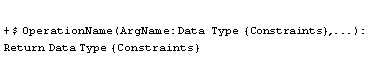Operation name: Required Any number of arguments is allowed Return data type: Required for a return value, but return values themselves are optional Visibility: Required before code generation Class operation: Optional Argument name: Required for each argument, but arguments themselves are optional Argument data type: Required for each argument, but arguments themselves are optional Constraints: Optional
operation Name Arguments Return DataType

### Operation definitions

Let's create an example operation to determine the total amount due on an order. The total is the sum of all line items less the volume discount. Each line item amount is the product of the unit price and discount. We need the answer back as a dollar amount.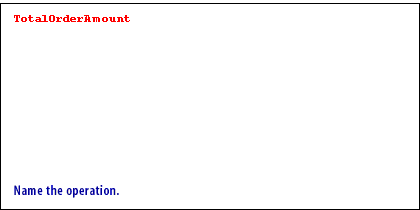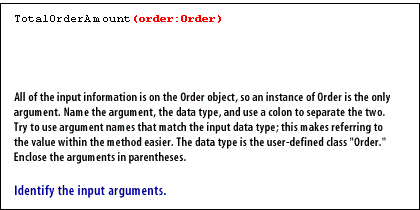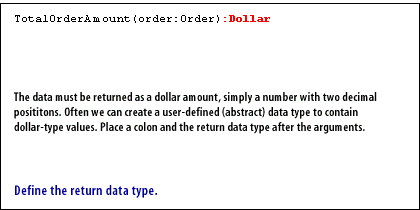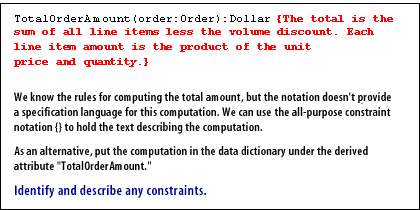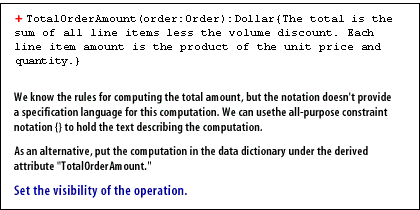1. Name the operation
2. Identify the input arguments. All of the input information is on the Order object, so an instance of Order is the only argument. Name the argument, the data type, and use a colon to a separate the two.
3. Define the return data type. The data must be returned as a dollar amount, simply a number with two decimal positions.
4. Identify and describe any constraints. We know the rules for computing the total amount, but the notation does not provide a specification language for this computation.
5. Set the visibility of the operation. We know the rules for computing the total amount, but the notation does not provide a specification language for this computation.We can use the all-purpose constraint notation() to hold the text describing the computation.

Total Order Amount Modeling Operations. In the next lesson, you will learn how to model classes using attributes and operations.

### Modeling Operations - Quiz

Click the Quiz link below to take a short multiple-choice quiz on modeling operations.
Modeling Operations - Quiz